# Maharashtra Board Practice Set 29 Class 6 Maths Solutions Chapter 11 Ratio-Proportion

Balbharti Maharashtra State Board Class 6 Maths Solutions covers the Std 6 Maths Chapter 11 Ratio-Proportion Class 6 Practice Set 29 Answers Solutions.

## 6th Standard Maths Practice Set 29 Answers Chapter 11 Ratio-Proportion

Question 1.
If 20 metres of cloth costs Rs 3600, find the cost of 16 m of cloth.
Solution:
Cost of 20 metres of cloth = Rs 3600
∴ Cost of 1 metre of cloth = $$\frac{\text { cost of } 20 \text { metres of cloth }}{20}=\frac{3600}{20}$$
= Rs 180
∴ Cost of 16 metres of cloth = Cost of 1 metre of a cloth × 16
= 180 x 16 = Rs 2880
∴ The cost of 16 metres of cloth is Rs 2880.

Question 2.
Find the cost of 8 kg of rice, if the cost of 10 kg is Rs 325.
Solution:
Cost of 10 kg rice = Rs 325
∴ Cost of 10 kg rice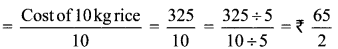Cost of 8 kg rice = Cost of 1 kg rice x 8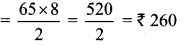∴ The cost of 8 kg rice is Rs 260.

Question 3.
If 14 chairs cost Rs 5992, how much will have to be paid for 12 chairs?
Solution:
Cost of 14 chairs = Rs 5992
∴ Cost of 1 chairs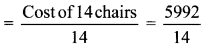= Rs 428
∴ Cost of 12 chairs = Cost of 1 chair x 12
= 428 x 12 = Rs 5136
∴ The amount to be paid for 12 chairs is Rs 5136.

Question 4.
The weight of 30 boxes is 6 kg. What is the weight of 1080 such boxes?
Solution:
Weight of 30 boxes = 6 kg
∴ Weight of 1 box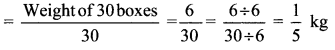∴ Weight of 1080 boxes = Weight of 1 box x 1080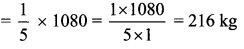∴ The weight of 1080 boxes is 216 kg.

Question 5.
A car travelling at a uniform speed covers a distance of 165 km in 3 hours. At that same speed,
a. How long will it take to cover a distance of 330 km?
b. How far will it travel in 8 hours?
Solution:
Distance covered in 3 hours = 165 km
Distance covered in 1 hour= 55 km
a. Time required to covered a distance of 330 km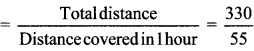= 6 hours
∴ The time required to cover a distance of 330 km is 6 hours.

b. Distance traveled in 8 hours = Distance covered in 1 hour x 8
= 55 x 8 = 440 km
∴ The distance traveled in 8 hours is 440 km.

Question 6.
A tractor uses up 12 litres of diesel while ploughing 3 acres of land. How much diesel will be needed to plough 19 acres of land?
Solution:
Diesel required to plough 3 acres of land =12 litres
∴ Diesel required to plough 1 acre of land= 4 liters
∴ Diesel required to plough 19 acres of land = Diesel required to plough 1 acre of land x 19
= 4 x 19 = 76 litres
∴ Diesel needed to plough 19 acres of land is 76 litres.

Question 7.
At a sugar factory, 5376 kg of sugar can be obtained from 48 tonnes of sugarcane. If Savitatai has grown 50 tonnes of sugarcanes, how much sugar will it yield?
Solution:
Sugar obtained from 48 tonnes of sugarcane = 5376 kg
∴ Sugar obtained from 48 tonnes of sugarcane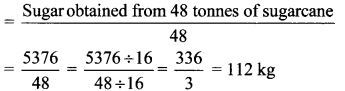∴ Sugar obtained from 50 tonnes of sugarcane = Sugar obtained from 1 tonne of sugarcane x 50
= 112 x 50 = 5600 kg
∴ 50 tonnes of sugarcane will yield 5600 kg of sugar.

Question 8.
In an orchard, there are 128 mango trees in 8 rows. If all the rows have an equal number of trees, how many trees would there be in 13 rows?
Solution:
Number of mango trees in 8 rows =128
Number of mango trees in 1 row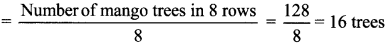∴ Number of mango trees in 13 rows = Number of mango trees in 1 row x 13
= 16 x 13 = 208
∴ The number of mango trees in 13 rows are 208.

Question 9.
A pond in a field holds 120000 litres of water. It costs Rs 18000 to make such a pond. How many ponds will be required to store 480000 litres of water, and what would be the expense?
Solution:
Capacity of 1 pond = 1,20,000 litres
Total quantity of water = 4,80,000 litres
∴ Number of ponds required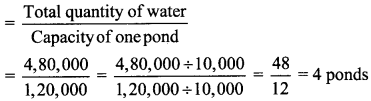Amount required to make 1 pond = Rs 18,000
∴ Amount required to make 4 ponds = Amount required to make 1 pond x 4
= 18,000 x 4 = Rs 72,000
∴ The number of ponds required to store 4,80,000 litres of water is 4, and the expense incurred in making the ponds is Rs 72,000.

#### Maharashtra Board Class 6 Maths Chapter 11 Ratio-Proportion Practice Set 29 Intext Questions and Activities

Question 1.
Vijaya wanted to gift pens to seven of her friends on her birthday. When she went to a shop to buy them, the shopkeeper told her the rate for a dozen pens.
i. Can you help Vijaya to find the cost of 7 pens?
ii. If you find the cost of one pen, you can also find the cost of 7, right? (Textbook pg. no. 59)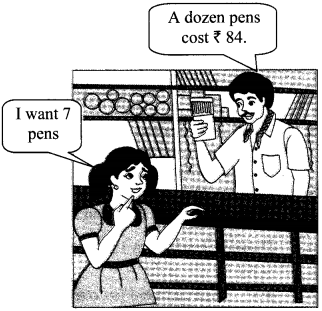Solution:
Cost of 12 pens = Rs 84.
∴ Cost of 12 pens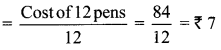∴ Cost of 7 pens = Cost of one pen x Number of pens = 7 × 7
∴ Cost of 7 pens = Rs 49
∴ The cost of 7 pens (Rs 49) can be found by unitary method.